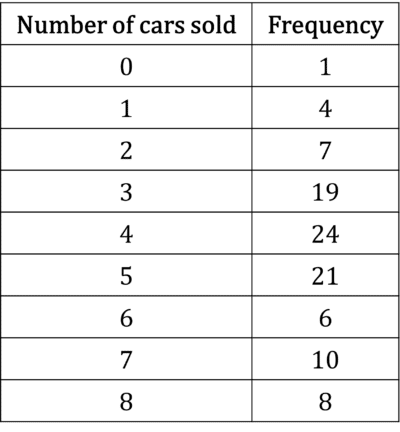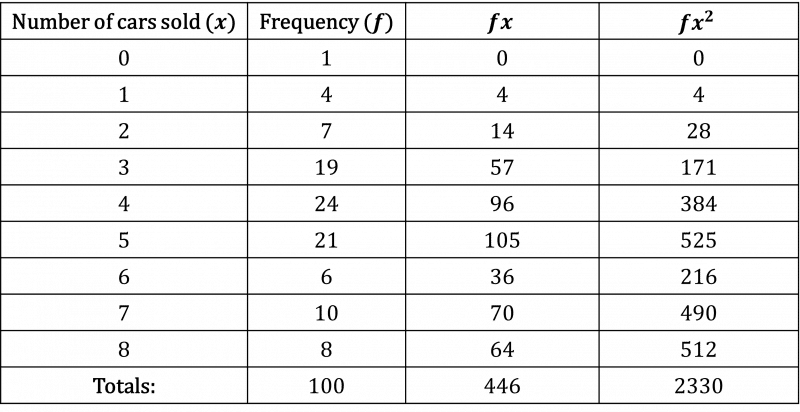# Mean and Standard Deviation

A LevelAQAEdexcelOCR

## Mean and Standard Deviation

Mean, median and mode are averages of data sets – known as measures of central tendency. Range is a measure of the spread of the data. We can also analyse the spread of the data in more sophisticated ways, such as standard deviation.

A LevelAQAEdexcelOCR

## Recap: Mean, Median, Mode and Range

The mean is the sum of the data points divided by the total number of data points. The median is the middle value of the data points when they are ordered (or the midpoint between the two middle values if there are an even number of data points). The mode is the value that appears the most often. The range is the difference between the highest and the lowest value. The interquartile range is the range after we discard the top and bottom quarters of the data.

Notation for the mean: The mean is often written as $\bar{x}=\dfrac{\sum{x}}{n}$ where $x$ is each data point, or $\bar{x}=\dfrac{\sum{fx}}{\sum{f}}$ where $x$ is a data value and $f$ is the frequency of that data value.

A LevelAQAEdexcelOCR

## Variance and Standard Deviation

Variance, like range, is a measure of the spread of the data. However, variance is a more intelligent measure than range as it takes all of the data into account. The formula for variance looks a little scary:

$\dfrac{\sum{(x-\bar{x})^{2}}}{n}$ or $\dfrac{\sum{x^{2}}}{n}-\bar{x}^{2}$ or $\dfrac{\sum{fx^{2}}}{\sum{f}}-\bar{x}^{2}$

It is easier to remember a simple rule:

Variance is (mean of the squares) – (square of the mean)

Standard deviation is the square root of the variance.

$\text{standard deviation}=\sqrt{\text{variance}}$

A LevelAQAEdexcelOCR

## Notation for Mean and Variance

The mean can be written as $\mathbb{E}(x)$, which means the expectation or expected value of $x$.

Note: the mean of the squares is written as $\mathbb{E}(x^{2})$, so the variance is $\mathbb{E}(x^{2})-(\mathbb{E}(x))^{2}$

The standard deviation is often written as the Greek letter $\sigma$, which means that the variance can be written as $\sigma^{2}$. You may also see the variance written as $\text{var}(x)$.

We can also use Sxy notation:

$S_{xy}=\sum{(x-\bar{x})(y-\bar{y})}=\sum{xy}-\dfrac{\sum{x}\sum{y}}{n}$

Using this notation, the variance of $x$ is equal to $\dfrac{S_{xx}}{n}$.

A LevelAQAEdexcelOCR
A LevelAQAEdexcelOCR

## Example 1: Finding the Mean and Standard Deviation

Find the mean and standard deviation of $6,10,4,7,24,13,9,15$.

[3 marks]

\begin{aligned}\text{mean}&=\dfrac{1}{8}(6+10+4+7+24+13+9+15)\\[1.2em]&=11\end{aligned}

Then to find the standard deviation,

\begin{aligned}&\text{mean of squares}\\[1.2em]&=\dfrac{1}{8}(6^{2}+10^{2}+4^{2}+7^{2}+24^{2}+13^{2}+9^{2}+15^{2})\\[1.2em]&=\dfrac{1}{8}(36+100+16+49+576+169+81+225)\\[1.2em]&=156.5\end{aligned}

\begin{aligned}\text{square of mean}&=11^{2}\\[1.2em]&=121\end{aligned}

\begin{aligned}\text{variance}&=\text{mean of squares}-\text{square of mean}\\[1.2em]&=156.5-121\\[1.2em]&=35.5\end{aligned}

\begin{aligned}\text{standard deviation}&=\sqrt{\text{variance}}\\[1.2em]&=\sqrt{35.5}\\[1.2em]&=5.96\end{aligned}

A LevelAQAEdexcelOCR

## Example 2: Standard Deviation From Grouped Frequency Table

The number of cars sold by $100$ small used car dealerships was monitored over the course of a day. The results have been collated into a table.What is the mean and standard deviation of the number of cars sold?

[6 marks]

To start, set up a table like this:\begin{aligned}\text{mean}&=\bar{x}\\[1.2em]&=\dfrac{\sum{fx}}{\sum{f}}\\[1.2em]&=\dfrac{446}{100}\\[1.2em]&=4.46\end{aligned}

\begin{aligned}\text{variance}&=\dfrac{\sum{fx^{2}}}{\sum{f}}-\bar{x}^{2}\\[1.2em]&=\dfrac{2330}{100}-4.46^{2}\\[1.2em]&=23.3-19.8916\\[1.2em]&=3.4084\end{aligned}

$\text{standard deviation} = \sqrt{3.4084} = 1.846$ ($3$ dp)

A LevelAQAEdexcelOCR

## Mean and Standard Deviation Example Questions

a)

\begin{aligned}\text{mean}&=\dfrac{10+4+7+11+3}{5}\\[1.2em]&=7\end{aligned}

b) For the median we must order the data:

$3,4,7,10,11$

We can see that the middle value is $7$, so the median is $7$

c)

\begin{aligned}\text{variance}&=\dfrac{10^{2}+4^{2}+7^{2}+11^{2}+3^{2}}{5}-7^{2}\\[1.2em]&=\dfrac{100+16+49+121+9}{5}-49\\[1.2em]&=\dfrac{295}{5}-49=59-49\\[1.2em]&=10\end{aligned}

$\sigma=9.2$

\begin{aligned}\text{variance}&=\sigma^{2}\\[1.2em]&=9.2^{2}\\[1.2em]&=84.64\end{aligned}

$\text{variance}=\text{mean of squares}-\text{square of mean}$

$84.64=\text{mean of squares}-5^{2}$

$84.64=\text{mean of squares}-25$

\begin{aligned}\text{mean of squares}&=25+84.64\\[1.2em]&=109.64\end{aligned}

Set up the table as in the second example:\begin{aligned}\text{mean}&=\bar{x}\\[1.2em]&=\dfrac{\sum{fx}}{\sum{f}}\\[1.2em]&=\dfrac{185}{30}\\[1.2em]&=6.17\end{aligned}

\begin{aligned}\text{variance}&=\dfrac{\sum{fx^{2}}}{\sum{f}}-\bar{x}^{2}\\[1.2em]&=\dfrac{1265}{30}-6.17^{2}\\[1.2em]&=42.2-38.0\\[1.2em]&=4.14\end{aligned}

A Level

A Level

## You May Also Like...### MME Learning Portal

Online exams, practice questions and revision videos for every GCSE level 9-1 topic! No fees, no trial period, just totally free access to the UK’s best GCSE maths revision platform.

£0.00